# Sectional curvature

This article defines a notion of curvature for a differential manifold equipped with a Riemannian metric

The equivalent notion for a pseudo-Riemannian manifold is: Sectional curvature for a pseudo-Riemannian manifold

## Definition

### Given data

A Riemannian manifold$(M,g)$ viz a differential manifold$M$ equipped with a Riemannian metric$g$.

### Definition part

Let$\Pi$ be a tangent plane to$M$ at a point$p \in M$. Then, the sectional curvature of$\Pi$ at$p$ is defined as follows: take two linearly independent vectors$X$ and$Y$ in$\Pi$, and calculate:$g(R(X,Y)Y,X)$

viz the inner product of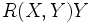$R(X,Y)Y$ and$X$ with respect to$g$.

Divide this by the square of the area of the parallelogram formed by$X$ and$Y$. This ratio defines the sectional curvature of$\Pi$, denoted as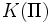$K(\Pi)$.

Here is the more explicit formula:$\frac{g(R(X,Y)Y,X)}{g(X,X)g(Y,Y) - g(X,Y)^2}$

Note that the denominator cannot vanish because$X$ and$Y$ are independent vectors.

### For a pseudo-Riemannian manifold

Further information: Sectional curvature for a pseudo-Riemannian manifold

We can also define the sectional curvature of a pseudo-Riemannian manifold. The same definition works.

## Facts

### Sectional curvature determines Riemann curvature tensor

This is analogous to the fact that Ricci curvature determines the Ricci curvature tensor

The following facts are true about the Riemann curvature tensor:

• The Riemann curvature tensor can be viewed as a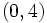$(0,4)$-tensor: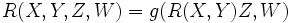$R(X,Y,Z,W) = g(R(X,Y)Z,W)$

• The above map is antisymmetric in$X, Y$ (For full proof, refer: Antisymmetry of Riemann curvature tensor and antisymmetric in$Z$ and$W$. Further it is symmetric in the pairs$(X,Y)$ and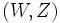$(W,Z)$. Hence, it is a symmetric bilinear form on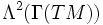$\Lambda^2(\Gamma(TM))$.

Thus, given the sectional curvature for all tangent planes, we can back-calculate the Riemann curvature tensor from it by the usual polarization trick. The idea is to view the Riemann curvature tensor as a symmetric bilinear form on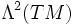$\Lambda^2(TM)$, and the sectional curvature as the values that this form takes on pairs$(\Pi,\Pi)$ of (special kinds of) unit vectors in$\Lambda^2(\Gamma(TM))$.